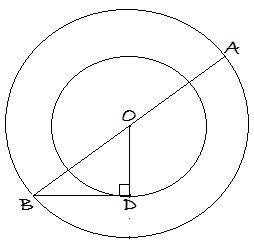# A Weird Cut Of Concentric Circles

Geometry Level 3In the figure above, the circles are concentric with centre $O$. $B$ is a point on the larger circle. $D$ is a point on the smaller circle. $BD$ is joined, provided that $BD$ touches the circle at only one point. Now $OB$ is joined and extended to meet the larger circle at $A$. Find the distance between points $A$ and $D$ if the radii of the circles are $4\sqrt{13}$ and $8$ units respectively.

Clarification: Concentric circles are circles with a common centre.

×